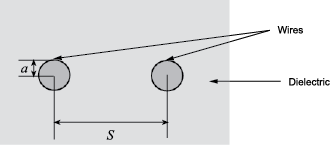txlineTwoWire

Create two-wire transmission line

Description

Use the txlineTwoWire object to create a two-wire transmission line. A cross-section of a two-wire transmission line is shown in this figure. The physical characteristics of a two-wire transmission line include the radii of the conducting wires, a, the separation or physical distance between the wire centers, S, and the relative permittivity and permeability of the wires. RF Toolbox™ software assumes that the relative permittivity and permeability are uniform.Creation

Description

twowiretxline = txlineTwoWire creates a default two-wire transmission line object.

example

twowiretxline = txlineTwoWire(Name,Value) sets Properties using one or more name-value pairs. For example, txline = txlineTwoWire('Separation',0.0046) creates a two-wire transmission line with a dielectric thickness of 0.0046 meters.

Properties

expand all

Name of the two-wire transmission line, specified as a string scalar or a character vector.

Example: 'Name','twowire1'

Example: twowiretxline.Name = 'twowire1'

Data Types: char | string

Physical length of the transmission line, specified as a positive scalar in meters.

Example: 'LineLength',0.0200

Example: twowiretxline.LineLength = 0.0200

Data Types: double

Conducting wire radius in the two-wire transmission line, specified as a positive scalar in meters.

Data Types: double

Thickness of the dielectric, specified as a positive scalar in meters.

Example: 'Separation',0.0025

Example: twowiretxline.Separation = 0.0025

Data Types: double

Relative permeability of the dielectric, specified as a positive scalar. Relative permeability of the dielectric, μ, to the permeability in free space, μ0.

Example: 'MuR',1.5

Example: twowiretxline.MuR = 1.5

Data Types: double

Relative permittivity of the dielectric, specified as a positive scalar.

Example: 'EpsilonR',3.3

Example: twowiretxline.EpsilonR = 3.3

Data Types: double

Loss angle tangent of the dielectric, specified as a nonnegative scalar in degrees

Example: 'LossTangent',1

Example: twowiretxline.LossTangent = 1

Data Types: double

Conductivity of the conductor, specified as a scalar in Siemens per meter (S/m).

Example: 'SigmaCond',2

Example: twowiretxline.SigmaCond = 2

Data Types: double

Stub transmission line termination, specified as 'NotApplicable', 'Open' or 'Short'.

Example: 'Termination','Short'

Example: twowiretxline.Termination = 'Short'

Data Types: char

Type of stub, specified as 'NotAStub', 'Series' or 'Shunt'.

Example: 'StubMode','Series'

Example: twowiretxline.StubMode = 'Series'

Data Types: char

Number of input and output ports, specified as a positive scalar.

Data Types: double

Terminals of coaxial transmission line, specified as a cell array of strings.

Data Types: char | string

Object Functions

 sparameters Calculate S-parameters for RF data, network, circuit, and matching network objects groupdelay Group delay of S-parameter object or RF filter object or RF Toolbox circuit object noisefigure Calculate noise figure of transmission lines, series RLC, and shunt RLC circuits getZ0 Calculate characteristic impedance with and without dispersion for transmission line circuit Circuit object clone Create copy of existing circuit element or circuit object

Examples

collapse all

Create a two-wire transmission line using these specifications:

• Dielectric - air

• Thickness of dielectric or separation - 1.088 mm

• Permittivity or EpsilonR - 1.0054